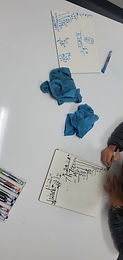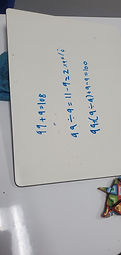Mr. Jake

Target 1​

Lesson Type:

Continuation

Measurment

:

Techniques and Formulas

Calculate circle measurements.

1:

Identify Pi (π) as a constant of all circles.

2:

Determine that Pi (π) is a ratio of circumference to diameter.

3:

Explain that Pi (π) is a special number, that continues into infinity without repeating.

4:

Recognize that Pi (π) is generally represented by 3.14.

5:

Calculate the diameter and circumference of a circle when given the radius (and vice versa).

7th

Vocabulary:

Activities:

Practiced measuring circular objects to find circle measurements.

Used different circle formulas to calculate circle measurementsHome Exploration

Guiding Questions:Absent Students:

Aaron

Target 2

:

1:

Explain the process for determining where to place the decimal point in the quotient of a decimal problem.

2:

Insert zeros where necessary when solving decimal division problems.

3:

Define the role that place-value plays in decimal computation.

4:

Utilize the standard algorithm to divide decimal numbers.

5:

Express that there will be no remainders in decimal division.

7th

Vocabulary:

Decimal, Repeated

Activities:

Discussed the process for dividing decimal numbers and dealing with repeated decimals

Determined how to use long division to get decimal and fractional answersHome Exploration

Guiding Questions:Target 3

:

1:

Use the given information in a problem to identify the correct answer.

2:

Use the given information to determine which is not the correct answer.

3:

Think outside of the box to solve problems.

4:

Follow given rules to solve problems.

7th

Vocabulary:

Activities:

Solved logical puzzles involving arithmetic and spatial reasoningHome Exploration